Author: Oscar Cronquist Article last updated on May 04, 2022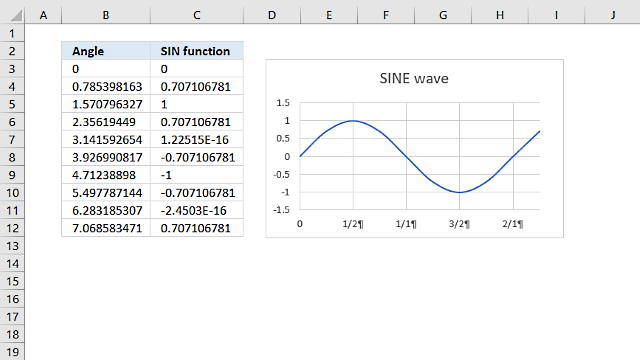The SIN function calculates the sine of an angle.

Formula in cell C3:

=SIN(B3)

SIN(number)

### Arguments

 number Required. The radian angle you want to know the sine of.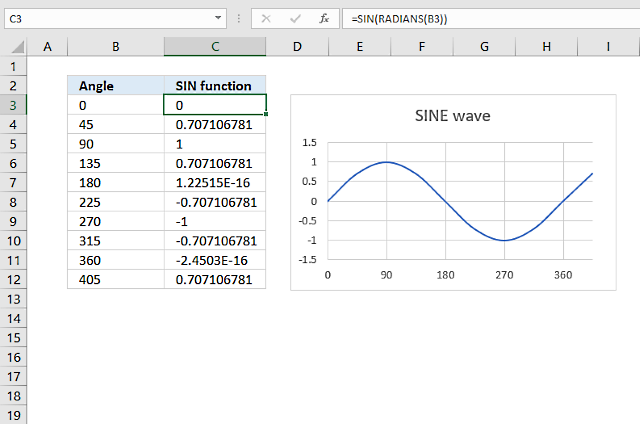### How to plot a sine wave or sinusoidal wave on a chart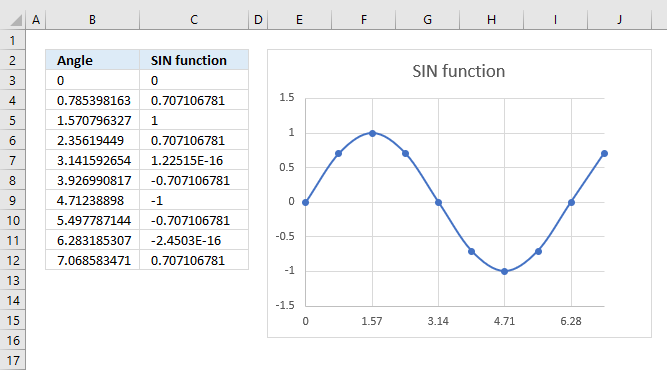The steps below describe in great detail how to graph a sine wave in an Excel chart.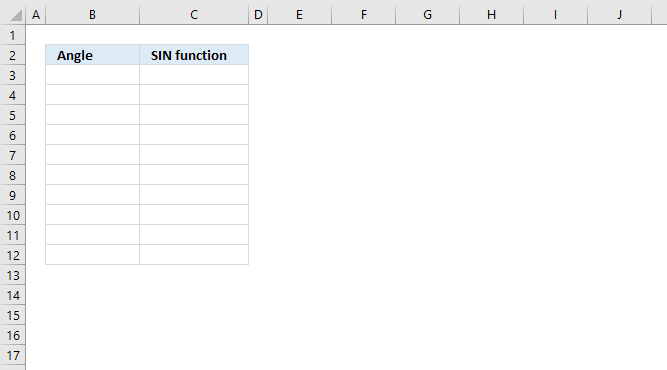The SIN function requires you to use radians in the function argument. Select cell B3 and type 0 (zero) then press Enter.

Type in cell B4:

=PI()*(ROW(A1)/4)

Press Enter. Copy cell B4 and paste to cells below as far as needed.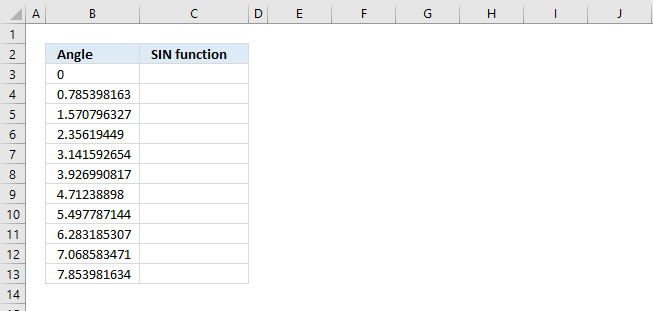Select cell C3. Type

=SIN(B3)

Press Enter.

#### How to quickly copy a formula and paste to cells below

Double press with left mouse button on the black dot in the lower right cell corner, see the animated image below.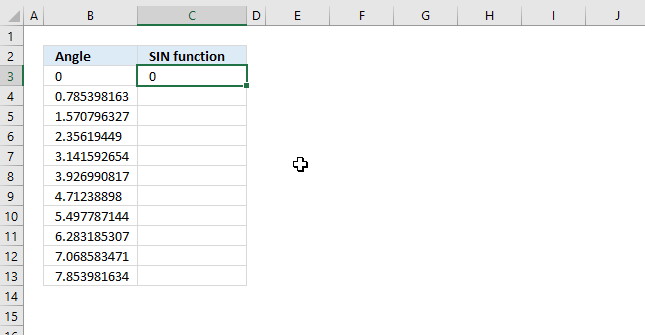This will copy cell C3 to cells below as far as needed based on values in adjacent column B.

#### How to insert a Scatter chart with Smotth Lines and Markers

Select cell range B3:C12 and go to tab "Insert" on the ribbon.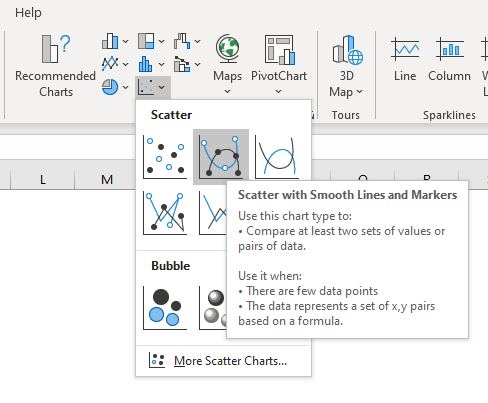Press with left mouse button on the "Scatter" button, a pop-up menu appears. Press with left mouse button on "Scatter with Smooth Lines and Markers" button, see image above.

#### How to reposition a chart?

The chart appears on your current worksheet, press and hold with the left mouse button on the chart. Drag with mouse to move the chart to the desired location. Release the left mouse button when the new location is reached.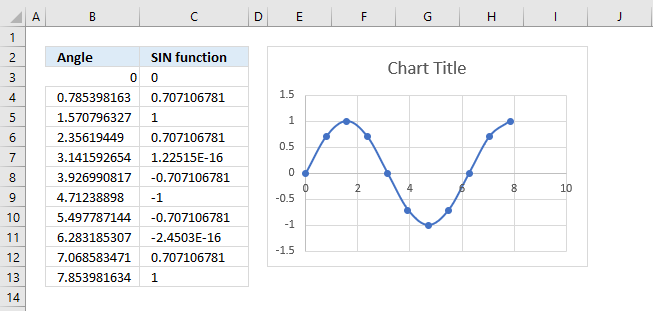#### How to change the x-axis location in an Excel chart?

Doublepress with left mouse button on the y-axis to quickly show axis chart settings.Press with left mouse button on the radio button "Axis value" below "Horizontal axis crosses". Type -1.5 in the field next to the radio button, see image above. Press Enter. This moves the x-axis below the gridlines.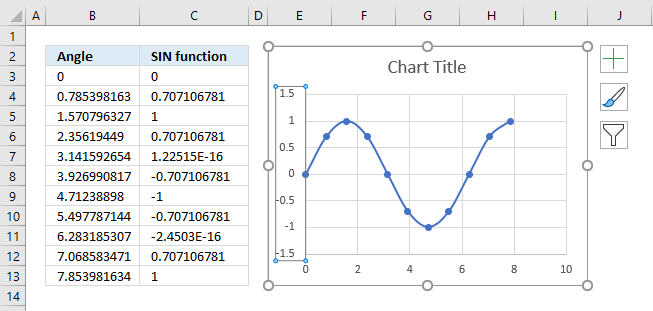#### How to change the chart x-axis range?

The x-axis ranges from 0 to 10, if you want to only show values from 0 to the last value in column B then copy cell B13.

Double press with left mouse button on the y-axis to open the axis settings.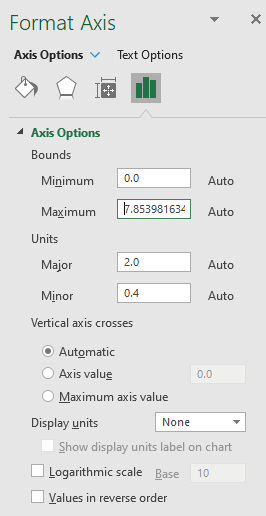Type the maximum number you want to be shown in Bounds - Maximum, in this case, paste the value you copied in the previous step.

#### Explaining formula in cell B4

I recommend the "Evaluate Formula" tool when trying to understand or troubleshoot Excel formulas. Select cell B4.

Go to tab "Formulas" on the ribbon, then press with left mouse button on the "Evaluate Formula" button. The following dialog box appears, see image below.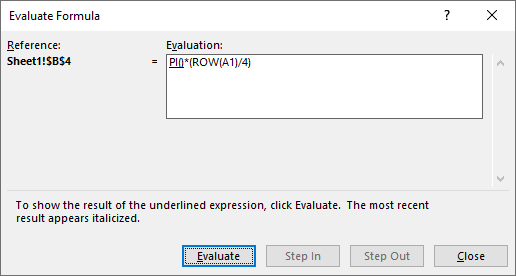The dialog box allows you to see the formula and the part that is going to be evaluated (underlined expression).  The most recent calculation is italicized, however, the image above shows the first calculation step. No expression is italicized because there has not been any calculation yet.

Press with left mouse button on the "Evaluate" button located on the dialog box to calculate the next part of the formula.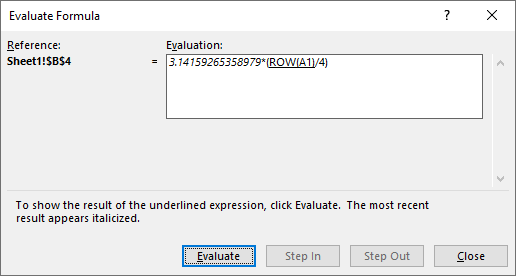The image above shows the result of PI() italicized and ROW(A1) is underlined meaning it will be calculated in the next step.

##### Step 1 - Create a sequence

The ROW function allows you to create a sequence or series of numbers based on a relative cell reference, in this case, A1.

A relative cell reference changes when you copy the cell and paste it to another cell. This makes the formula return a new value in each cell.

The ROW function returns the row number from a cell reference. Cell A1 is on row 1.

ROW(A1)

returns 1.

##### Step 2 - Divide with 4

This step divides the row number with four.

ROW(A1)/4

becomes

1/4

and returns 0.25.

##### Step 3 - Multiply with pi

The PI function returns the PI number.

PI()*(ROW(A1)/4)

becomes

PI()*0.25

becomes

3.141592654*0.25

and returns 0.785398163 in cell B4.

### How to change sine wave amplitude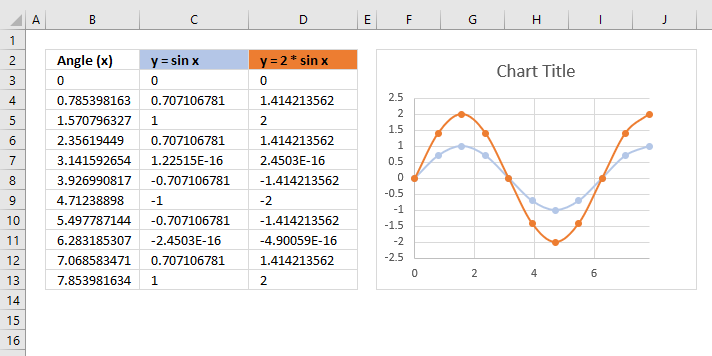The general form of the Sin wave is y = A*Sin(B*(x-C))+D

Constant A in the formula above changes the amplitude or height of the sine wave. The image above shows two different sine waves, a blue one and an orange one.

The orange sine wave has an amplitude or height of 2 and the blue one has an amplitude of 1.

Formula in cell C3:

=SIN(B3)

Formula in cell D3:

=2*SIN(B3)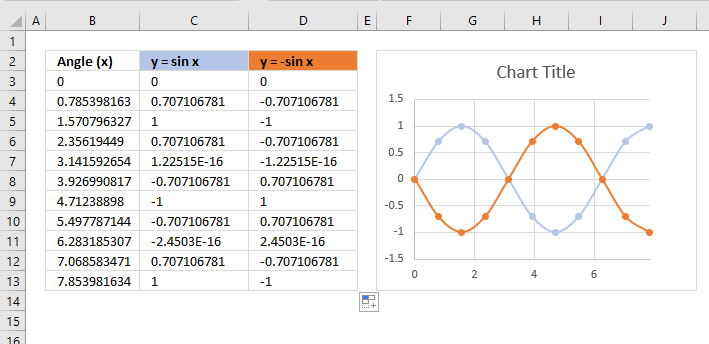The sine wave is mirrored if you change constant A to -1.

### How to change the sine wave period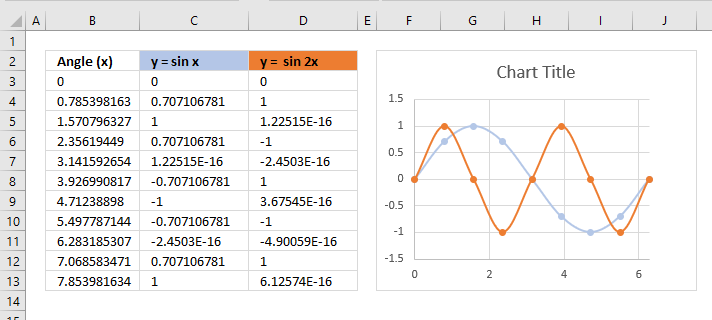The general form of the Sin wave is y = A*Sin(B*(x-C))+D

Constant B changes the period, the chart above shows two sine waves. The blue sine wave completes a cycle in 2π or 360°, however, the orange sine wave completes two cycles in 2π or 360°.

Formula in cell C3:

=SIN(B3)

Formula in cell D3:

=SIN(2*B3)

### How to change the sine wave midline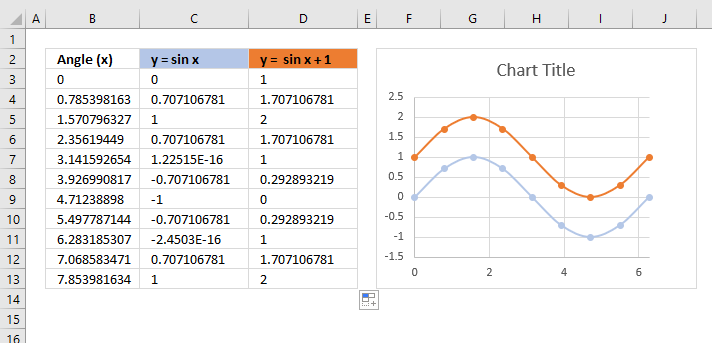The general form of the Sin wave is y = A*Sin(B*(x-C))+D

Constant D lets you change the midline which is the centerline in which the sine wave oscillates back and forth. The midline is horizontal and is right in between the minimum and maximum sine function values.

Formula in cell C3:

=SIN(B3)

The blue sine wave in column C has a maximum value of 1 and a minimum value of -1, the midline is 0 (zero).

Formula in cell D3:

=SIN(B3)+1

The orange sine wave in column D has a maximum value of 2 and a minimum value of 0, the midline is 1 (zero).

### How to use the SIN function with a right or right-angled triangle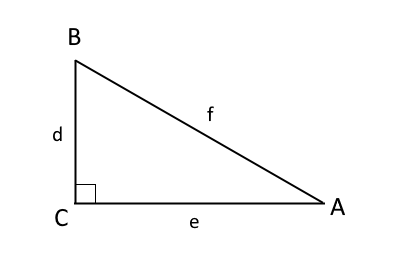A, B and C are angles, C is 90°, shown in the image above. A + B + C = 180°. One of the angles must be 90° (right angle triangle), in the example above C is 90°.

d, e, and f are lengths of the sides of the triangle. Side d and e are perpendicular to each other, meaning the angle between them are 90°.

SIN A = d/f

SIN A is equal to the ratio between sides d and f, this is only true if the triangle is a right-angled triangle.